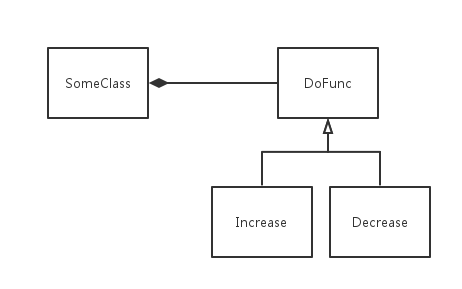完全不知道怎么取标题，但是不进来看看也许会后悔--其实我一开始想写关于virtual 函数的

class SomeClass
{
enum{ Length = 512};
int element[Length];
public:
void traver()
{
//做一些遍历
}
};

class DoFunc
{
public:
virtual ~DoFunc() {}
virtual void func(int&) = 0;
};

class Increase: public DoFunc
{
public:
void func(int& i)
{
++i;
}
};

class Decrease: public DoFunc
{
public:
void func(int& i)
{
--i;
}
};

class SomeClass
{
enum{ Length = 512};
int element[Length];
DoFunc* dofunc;

public:
SomeClass(DoFunc* indo): dofunc(indo) {}
void traver()
{
for(int i=0;i<Length;++i)
dofunc->func(element[i]);
}
void setFunc(DoFunc* indo)
{
dofunc = indo;
}
};


    Increase in;
Decrease de;
SomeClass s(&in);
//...
s.setFunc(&de);class Increase
{
public:
void operator () (int& i)
{
++i;
}
};

class Decrease
{
public:
void operator () (int& i)
{
--i;
}
};

class SomeClass
{
enum{ Length = 512};
int element[Length];
public:
SomeClass(){}
template<typename T>
void traver(T t)
{
for(int i=0;i<Length;++i)
t(element[i]);
}
};



    Increase in;
Decrease de;
SomeClass s;
s.traver(in);
s.traver(de);
s.traver([](int& i) -> void
{
i += 10;
});

[参考资料]
 Scott Meyers 著, 侯捷译. Effective C++ 中文版: 改善程序技术与设计思维的 55 个有效做法[M]. 电子工业出版社, 2011.
（条款35：考虑virtual 函数以外的选择）